Previous Page | Next Page

 The MCMC Procedure

## Example 52.2 Box-Cox Transformation

Box-Cox transformations (Box and Cox; 1964) are often used to find a power transformation of a dependent variable to ensure the normality assumption in a linear regression model. This example illustrates how you can use PROC MCMC to estimate a Box-Cox transformation for a linear regression model. Two different priors on the transformation parameter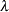are considered: a continuous prior and a discrete prior. You can estimate the probability ofbeing 0 with a discrete prior but not with a continuous prior. The IF-ELSE statements are demonstrated in the example.

### Using a Continuous Prior onThe following statements create a SAS data set with measurements of y (the response variable) and x (a single dependent variable):

```title 'Box-Cox Transformation, with a Continuous Prior on Lambda';
data boxcox;
input y x @@;
datalines;
10.0  3.0  72.6  8.3  59.7  8.1  20.1  4.8  90.1  9.8   1.1  0.9
78.2  8.5  87.4  9.0   9.5  3.4   0.1  1.4   0.1  1.1  42.5  5.1

... more lines ...

2.6  1.8  58.6  7.9  81.2  8.1  37.2  6.9
;
```

The Box-Cox transformation of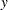takes on the form of:The transformed response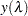is assumed to be normally distributed: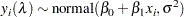The likelihood with respect to the original response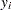is as follows: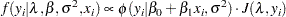where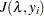is the Jacobian: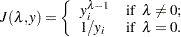And on the log-scale, the Jacobian becomes: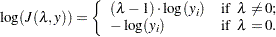There are four model parameters: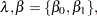and. You can considering using a flat prior onand a gamma prior on.

To consider only power transformations (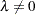), you can use a continuous prior (for example, a uniform prior from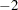to) on. One issue with using a continuous prior is that you cannot estimate the probability of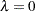. To do so, you need to consider a discrete prior that places positive probability mass on the point. See Modeling.

The following statements fit a Box-Cox transformation model:

```ods graphics on;
proc mcmc data=boxcox nmc=50000 thin=10 propcov=quanew seed=12567
monitor=(lda);

parms beta0 0  beta1 0  lda 1 s2 1;

beginnodata;
prior beta: ~ general(0);
prior s2 ~ gamma(shape=3, scale=2);
prior lda ~ unif(-2,2);
sd = sqrt(s2);
endnodata;

ys = (y**lda-1)/lda;
mu = beta0+beta1*x;
ll = (lda-1)*log(y)+lpdfnorm(ys, mu, sd);
model general(ll);
run;
```

The PROPCOV option initializes the Markov chain at the posterior mode and uses the estimated inverse Hessian matrix as the initial proposal covariance matrix. The MONITOR= option selectsas the variable to report. The ODS SELECT statement displays the summary statistics table, the interval statistics table, and the diagnostic plots.

The PARMS statement puts all four parameters,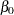,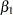,, and, in a single block and assigns initial values to each of them. Three PRIOR statements specify previously stated prior distributions for these parameters. The assignment to sd transforms a variance to a standard deviation. It is better to place the transformation inside the BEGINNODATA and ENDNODATA statements to save computational time.

The assignment to the symbol ys evaluates the Box-Cox transformation of y, where mu is the regression mean and ll is the log likelihood of the transformed variable ys. Note that the log of the Jacobian term is included in the calculation of ll.

Summary statistics and interval statistics for lda are listed in Output 52.2.1.

Output 52.2.1 Box-Cox Transformation
 Box-Cox Transformation, with a Continuous Prior on Lambda

The MCMC Procedure

Posterior Summaries
Parameter N Mean Standard
Deviation
Percentiles
25% 50% 75%
lda 5000 0.4702 0.0284 0.4515 0.4703 0.4884

Posterior Intervals
Parameter Alpha Equal-Tail Interval HPD Interval
lda 0.050 0.4162 0.5269 0.4197 0.5298

The posterior mean ofis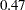, with a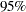equal-tail interval of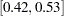and a similar HPD interval. The prefered power transformation would be
(roundingup to the square root transformation).

Output 52.2.2 shows diagnostics plots for lda. The chain appears to converge, and you can proceed to make inferences. The density plot shows that the posterior density is relatively symmetric around its mean estimate.

Output 52.2.2 Diagnostic Plots for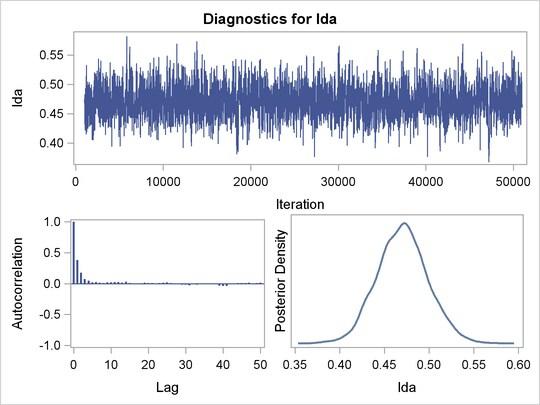To verify the results, you can use PROC TRANSREG (see Chapter 91, The TRANSREG Procedure ) to find the estimate of.

```proc transreg data=boxcox details pbo;
ods output boxcox = bc;
model boxcox(y / convenient lambda=-2 to 2 by 0.01) = identity(x);
output out=trans;
run;
ods graphics off;
```

Output from PROC TRANSREG is shown in Output 52.2.5 and Output 52.2.4. PROC TRANSREG produces a similar point estimate of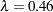, and the 95% confidence interval is shown in Output 52.2.5.

Output 52.2.3 Box-Cox Transformation Using PROC TRANSREG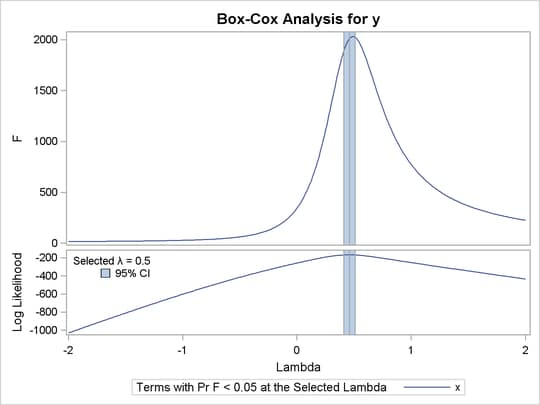Output 52.2.4 Estimates Reported by PROC TRANSREG
 Box-Cox Transformation, with a Continuous Prior on Lambda

The TRANSREG Procedure

Model Statement Specification Details
Type DF Variable Description Value
Dep 1 BoxCox(y) Lambda Used 0.5
Lambda 0.46
Log Likelihood -167.0
Conv. Lambda 0.5
Conv. Lambda LL -168.3
CI Limit -169.0
Alpha 0.05
Options Convenient Lambda Used
Ind 1 Identity(x) DF 1

The ODS data set bc contains the 95% confidence interval estimates produced by PROC TRANSREG. This ODS table is rather large, and you want to see only the relevant portion. The following statements generate the part of the table that is important and display Output 52.2.5:

```proc print noobs label data=bc(drop=rmse);
title2 'Confidence Interval';
where ci ne ' ' or abs(lambda - round(lambda, 0.5)) < 1e-6;
label convenient = '00'x ci = '00'x;
run;
```

The estimated 90% confidence interval is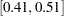, which is very close to the reported Bayesian credible intervals. The resemblance of the intervals is probably due to the noninformative prior that you used in this analysis.

Output 52.2.5 Estimated Confidence Interval onBox-Cox Transformation, with a Continuous Prior on Lambda Confidence Interval

Dependent Lambda R-Square Log Likelihood
BoxCox(y) -2.00   0.14 -1030.56
BoxCox(y) -1.50   0.17 -810.50
BoxCox(y) -1.00   0.22 -602.53
BoxCox(y) -0.50   0.39 -415.56
BoxCox(y) 0.00   0.78 -257.92
BoxCox(y) 0.41   0.95 -168.40 *
BoxCox(y) 0.42   0.95 -167.86 *
BoxCox(y) 0.43   0.95 -167.46 *
BoxCox(y) 0.44   0.95 -167.19 *
BoxCox(y) 0.45   0.95 -167.05 *
BoxCox(y) 0.46   0.95 -167.04 <
BoxCox(y) 0.47   0.95 -167.16 *
BoxCox(y) 0.48   0.95 -167.41 *
BoxCox(y) 0.49   0.95 -167.79 *
BoxCox(y) 0.50 + 0.95 -168.28 *
BoxCox(y) 0.51   0.95 -168.89 *
BoxCox(y) 1.00   0.89 -253.09
BoxCox(y) 1.50   0.79 -345.35
BoxCox(y) 2.00   0.70 -435.01

### ModelingWith a continuous prior on, you can get only a continuous posterior distribution, and this makes the probability of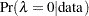equal toby definition. To consideras a viable solution to the Box-Cox transformation, you need to use a discrete prior that places some probability mass on the point 0 and allows for a meaningful posterior estimate of.

This example uses a simulation study where the data are generated from an exponential likelihood. The simulation implies that the correct transformation should be the logarithm andshould be 0. Consider the following exponential model: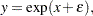where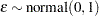. The transformed data can be fitted with a linear model: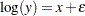The following statements generate a SAS data set with a gridded x and corresponding y:

```title 'Box-Cox Transformation, Modeling Lambda = 0';
data boxcox;
do x = 1 to 8 by 0.025;
ly = x + normal(7);
y = exp(ly);
output;
end;
run;
```

The log-likelihood function, after taking the Jacobian into consideration, is as follows: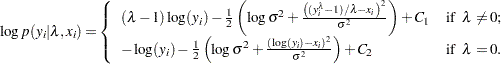where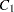and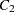are two constants.

You can use the function DGENERAL to place a discrete prior on. The function is similar to the function GENERAL, except that it indicates a discrete distribution. For example, you can specify a discrete uniform prior fromtousing

```prior lda ~ dgeneral(1, lower=-2, upper=2);
```

This places equal probability mass on five points,,
,,, and. This prior might not work well here because the grid is too coarse. To consider smaller values of, you can sample a parameter that takes a wider range of integer values and transform it back to thespace. For example, set alpha as your model parameter and give it a discrete uniform prior from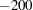to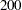. Then defineas alpha/100 socan take values betweenandbut on a finer grid.

The following statements fit a Box-Cox transformation by using a discrete prior on:

```proc mcmc data=boxcox outpost=simout nmc=50000 thin=10 seed=12567
monitor=(lda);

ods select PostSummaries PostIntervals;
parms s2 1 alpha 10;

beginnodata;
prior s2 ~ gamma(shape=3, scale=2);
if alpha=0 then lp = log(2);
else lp = log(1);
prior alpha ~ dgeneral(lp, lower=-200, upper=200);
lda = alpha * 0.01;
sd = sqrt(s2);
endnodata;

if alpha=0 then
ll = -ly+lpdfnorm(ly, x, sd);
else do;
ys = (y**lda - 1)/lda;
ll = (lda-1)*ly+lpdfnorm(ys, x, sd);
end;
model general(ll);
run;
```

There are two parameters, s2 and alpha, in the model. They are placed in a single PARMS statement so that they are sampled in the same block.

The parameter s2 takes a gamma distribution, and alpha takes a discrete prior. The IF-ELSE statements state that alpha takes twice as much prior density when it is 0 than otherwise. Note that on the original scale,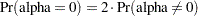. Translating that to the log scale, the densities become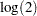and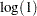, respectively. The lda assignment statement transforms alpha to the parameter of interest: lda takes values betweenand. You can model lda on a even smaller scale by dividing alpha by a larger constant. However, an increment of 0.01 in the Box-Cox transformation is usually sufficient. The sd assignment statement calculates the square root of the variance term.

The log-likelihood function uses another set of IF-ELSE statements, separating the case offrom the others. The formulas are stated previously. The output of the program is shown in Output 52.2.6.

Output 52.2.6 Box-Cox Transformation
 Box-Cox Transformation, Modeling Lambda = 0

The MCMC Procedure

Posterior Summaries
Parameter N Mean Standard
Deviation
Percentiles
25% 50% 75%
lda 5000 -0.00002 0.00201 0 0 0

Posterior Intervals
Parameter Alpha Equal-Tail Interval HPD Interval
lda 0.050 0 0 0 0

From the summary statistics table, you see that the point estimate foris 0 and both of the 95% equal-tail and HPD credible intervals are 0. This strongly suggests thatis the best estimate for this problem. In addition, you can also count the frequency ofamong posterior samples to get a more precise estimate on the posterior probability ofbeing 0.

The following statements use PROC FREQ to produce Output 52.2.7 and Output 52.2.8:

```ods graphics on;
proc freq data=simout;
ods select onewayfreqs freqplot;
tables lda /nocum plot=freqplot(scale=percent);
run;
ods graphics off;
```

Output 52.2.7 shows the frequency count table. An estimate ofis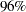. The conclusion is that the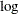transformation should be the appropriate transformation used here, which agrees with the simulation setup. Output 52.2.8 shows the histogram of.

Output 52.2.7 Frequency Counts ofBox-Cox Transformation, Modeling Lambda = 0

The FREQ Procedure

lda Frequency Percent
-0.0100 106 2.12
0 4798 95.96
0.0100 96 1.92

Output 52.2.8 Histogram of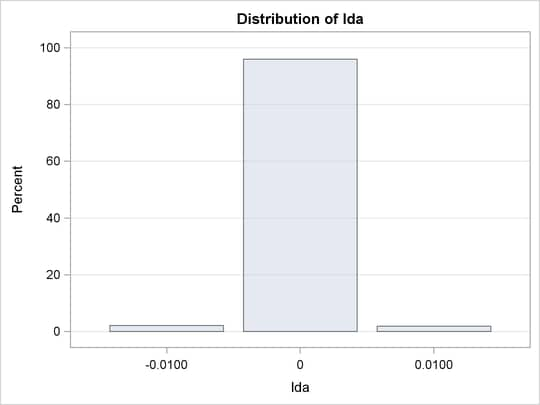Previous Page | Next Page | Top of Page